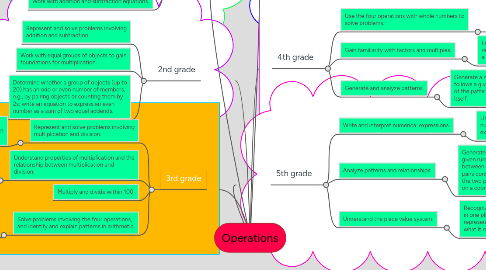# OperationsGet Started. It's FreeOperations## 2. kindergarten

### 4.1. Represent and solve problems involving multiplication and division.

4.1.1. nterpret products of whole numbers, e.g., interpret 5 × 7 as the total number of objects in 5 groups of 7 objects each.

### 4.2. Understand properties of multiplication and the relationship between multiplication and division.

4.2.1. Apply properties of operations as strategies to multiply and divide.

### 4.4. Solve problems involving the four operations, and identify and explain patterns in arithmetic.

4.4.1. Solve two-step word problems using the four operations. Represent these problems using equations with a letter standing for the unknown quantity

### 5.1. Use the four operations with whole numbers to solve problems.

5.1.1. Represent verbal statements of multiplicative comparisons as multiplication equations.

### 5.2. Gain familiarity with factors and multiples.

5.2.1. Find all factor pairs for a whole number in the range 1-100. Recognize that a whole number is a multiple of each of its factors

### 5.3. Generate and analyze patterns.

5.3.1. Generate a number or shape pattern that follows a given rule. Identify apparent features of the pattern that were not explicit in the rule itself.